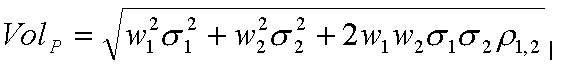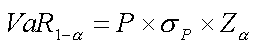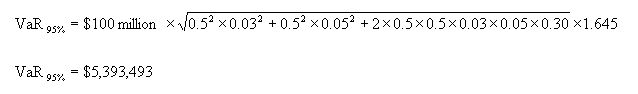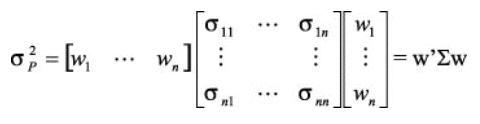# Analytical Approach to Calculating VaR (Variance-Covariance Method)

We earlier saw how VaR can be calculated using the parametric method. We will now look at this method in detail, and also understand how VaR can be easily calculated using matrices.

### VaR of a Single Asset

VaR of a single asset is the value of the asset multiplied by its volatility. Here, the volatility can be calculated at the desired confidence level.

An IBM stock is trading at $115 with a 1-year standard deviation of 20%. In the normal distribution, 95% confidence level is 1.645 standard deviations away from the mean. Therefore, our VaR at 95% confidence level will be: VaR (95%) = 115* 0.20 * 1.645 = 37.835 Assumption of Normality A distribution is described as normal if there is a high probability that any observation form the population sample will have a value that is close to the mean, and a low probability of having a value that is far from the mean. The normal distribution curve is used by many VaR models, which assume that asset returns follow a normal pattern. A VaR model uses the normal curve to estimate the losses that an institution may suffer over a given time period. Normal distribution tables show the probability of a particular observation moving a certain distance from the mean. If we look along a normal distribution table we see that at -1.645 standard deviations, the probability is 5%; this means that there is a 5% probability that an observation will be at least 1.645 standard deviations below the mean. This level is used in many VaR models. ### VaR of a Portfolio Generally VaR will not be calculated for a single position, but a portfolio of positions. In such a case will require the portfolio volatility. The portfolio volatility of a two-asset portfolio is given by:Where: • $w_{1}$ is the weighting of the first asset • $w_{2}$ is the weighting of the second asset • $\sigma_{1}$ is the standard deviation or volatility of the first asset • $\sigma_{2}$ is the standard deviation or volatility of the second asset • $\rho$ is the correlation coefficient between the two assets The VaR will then be given by:Where: • VaR (1 - $\alpha$) is the estimated VaR at the confidence level 100 × (1 - $\alpha$)%. • $Z_{\alpha}$ represents the no. of standard deviations on the left side of the mean, at the required standard deviation. ### VaR of a Portfolio - Example Let us assume that we want to calculate Parametric VaR at a 95% confidence level over a one-day horizon on a portfolio composed of two assets with the following assumptions: • P =$100 million
• $w_{1}$ = 50%
• $w_{2}$ = 50%
• $\sigma_{1}$ = 3%
• $\sigma_{2}$ = 5%
• $\rho$ = 30%### Portfolio of n Assets

If the portfolio is bigger than 2 assets, the volatility of the portfolio is expressed using matrix notation:Where:

• $w$ is the vector of the weights of the n assets.
• $w'$ is the transpose vector of $w$
• $\sum$ is the covariance matrix of the n assets

This is the reason why this method is also known as Variance Covariance method.

### Variance Covariance Method - Examples

The spreadsheet attached below contains two examples of calculating Value at Risk using the Variance Covariance (Parametric Method).

The first example is of a Two Asset Portfolio that takes the same example above and recalculates the VaR using the matrices.

The second example is of a Three Asset Portfolio where the VaR calculation is shown for a three-asset portfolio.

It is easy from there to expand the calculation to a portfolio of n assets. But be aware that you will soon reach the limits of Excel as we will have to calculate n(n-1)/2 terms for your covariance matrix.

#### Lesson Resources

This bundle contains spreadsheets to help calculate value-at-risk in excel.
Member-only